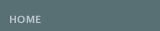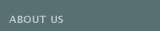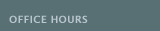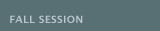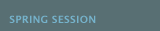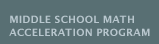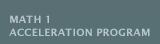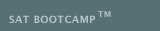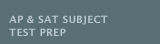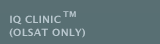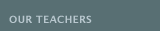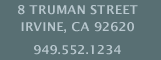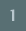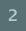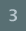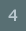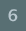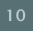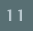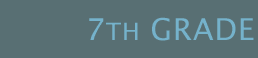The CRITICAL WRITING FOR THE 7th GRADE is a small group class that provides instruction aligned with the California's Common Core State Standards in the following areas: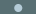Active Reading (Reading Comprehension): Students will read and understand 4-6 novels, cite several pieces of textual evidence to support inferences, determine a central theme and analyze its development over the course of the text, provide an objective summary, analyze how particular elements of a drama interact (e.g., how setting shapes the characters or plot), analyze how an author develops and contrasts the points of view (e.g., first and third person, limited and omniscient, subjective and objective) of different characters, and analyze recurring themes (e.g., the value of bravery, loyalty, and friendship, the effects of loneliness) in a text.Fundamentals of Effective Writing: Students will be writing clear, coherent, and focused essays to examine a thesis statement, introduce a claim, acknowledge and address alternate claims, support claims or counterarguments with logical reasoning and relevant evidence, use appropriate transitions to create cohesion and clarify relationships among ideas, establish a formal writing style and provide a concluding paragraph that supports the explanation presented. Students will be practicing the three writing genres (expository, persuasive arguments, and descriptive narratives) to include at least 500�800 words in each composition.Vocabulary Development: Students will integrate knowledge of individual words to enhance their writing, use knowledge of Greek, Latin, and Anglo-Saxon roots and affixes to understand content-area vocabulary, and clarify word meanings through the use of definition, example, restatement, or contrast.Grammar: For the grammar module of the class, students will learn to identify and use infinitives, participles, make clear references between pronouns and antecedents, explain the function of phrases and clauses, identify all parts of speech, types and structure of sentences, demonstrate the mechanics of writing, appropriate English usage and place modifiers properly and use the active voice. CAASPP test preparation will be integrated to formally prepare students specifically emphasizing the areas of reading comprehension, sentence structure, writing strategies and vocabulary. Some book purchases will be necessary. Students can expect homework and weekly quizzes. Grades will be given.
 E07A CRITICAL WRITING (7th Gr) January 27�June 5 (19 weeks)* *March 28�April 5 (Spring Recess)
 Wednesdays 4:00�6:00pm SHAGOURY
 MATH 7 ACCELERATED (MSMAP) is designed for the end of course (EOC) test for the 7th grade student for MATH 1 INTEGRATED placement in the 8th grade. This group class is aligned with the California's COMMON CORE State Standards:Ratios and Proportional Relationships: Use proportional relationships to solve multistep ratio and percent problems.Expressions and Equations: Use variables to represent quantities in a real-world or mathematical problem, and construct equations and inequalities to solve problems by reasoning about the quantities.Geometry: Use facts about supplementary, complementary, vertical, and adjacent angles in a multi-step problem to write and solve equations for an unknown angle in a figure. Solve real-world problems involving area, volume and surface area of 2 and 3 dimensional objects composed of triangles, quadrilaterals, polygons, cubes, and right prisms.Statistics and Probability: Investigate chance processes and develop, use, and evaluate probability models. Develop a probability model and use it to find probabilities of events.
 Students will be required to attend twice a week (one lesson to emphasize problem solving and word problems and the other to reinforce accuracy). Students are expected to complete additional exercise problem sets for homework. CAASPP test preparation will be integrated in the weekly lectures. Students can expect homework, quizzes, a mid-term and a final. Grades will be given.
 All seventh graders must first pass the end of year test in order to take Math 1 Integrated Acceleration Test in the Fall.
 M01A Math 7 Accel (Math 1 Integrated Placement-MSMAP)
 January 27�April 24 (13 weeks)* *February 17 (Holiday) *March 28�April 5 (Spring Recess)
 Mondays or Wednesdays 4:00-6:00pm FU
 January 27�June 5 (19 weeks)* *March 28�April 5 (Spring Recess)
 Thursdays 4:00-6:00pm FU
 MATH 8 (MSMAP) is designed for the end of course (EOC) test for MATH 1 INTEGRATED placement the following year. This course introduces the language and methods of algebra. Instruction will focus on 4 critical areas:Expressions and Equations: Solve real-world and mathematical problems of two linear equations in two variables algebraically, and estimate solutions by graphing the equations.Functions: Interpret the equation y=mx+b as defining a linear function. Construct a function to model a linear relationship between two quantities.Geometry: Apply the Pythagorean Theorem to determine unknown side lengths in right triangles in real-world and mathematical problems in two and three dimensions. Apply the formulas for the volumes of cones, cylinders, and spheres and use them to solve real-world and mathematical problems.Statistics and Probability: Construct and interpret scatter plots for bivariate measurement data to investigate patterns of association between two quantities. Students will be required to attend twice a week (one lesson to emphasize problem solving and word problems and the other to reinforce accuracy). Students are expected to complete additional exercise problem sets for homework. CAASPP test preparation will be integrated in the weekly lectures. Students can expect homework, quizzes, a mid-term and a final. Grades will be given.
 M01A MATH 8 (MATH 1 INT PLACEMENT-MSMAP)
 January 27�April 24 (13 weeks)* *February 17 (Holiday) *March 28�April 5 (Spring Recess)
 Wednesdays 4:00-6:00pm CHAALAN
 January 27�June 5 (19 weeks)* *February 17 (Holiday) *March 28�April 5 (Spring Recess) *May 25 (Holiday)
 Mondays 4:00-6:00pm CHAALAN
 In MATH 1 at the BARUN ACADEMIC CENTER, students will learn to reason abstractly and quantitatively. Instruction will highlight 4 critical areas:Algebra: Represent and solve equations and inequalities graphically. Explain why the x-coordinates of the points where the graphs of the equations y=f(x) and y=g(x) intersect are the solutions of the equation f(x)=g(x). Include cases where f(x) and/or g(x) are linear, polynomial, rational, absolute value, exponential, and logarithmic functions.Functions: Calculate and interpret the average rate of change of a function (presented symbolically or as a table) over a specified interval. Estimate the rate of change from a graph.Geometry: Develop definitions of rotations, reflections, and translations in terms of angles, circles, perpendicular lines, parallel lines, and line segments. Prove the slope criteria for parallel and perpendicular lines and use them to solve geometric problems.Statistics and Probability: Summarize categorical data for two categories in two-way frequency tables. Fit a function to the data. This group class is for the student enrolled in Math 1 (Integrated). Students can expect homework, quizzes, a mid-term and a final. Grades will be given.
 M02A MATH 1 (INTEGRATED) January 27�June 5 (19 weeks)* *February 17 (Holiday) *March 28�April 5 (Spring Recess) *May 25 (Holiday)
 Mondays 4:00-6:00pm CHANG